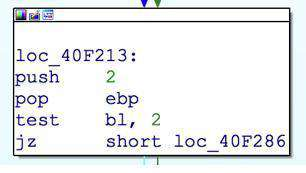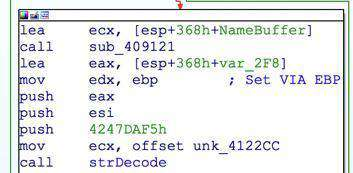# Using concolic execution for static analysis of malwareReverse engineering is about reducing the complex equation of binary code into na abstract understandable form . Dynamic and static analysis can speed up the process to a large extent , but they have their limitations when malware further tries to obfuscate and add an extra layer of protection to make analysis harder . Hiding import calls , obfuscated strings , use of visualized code are some of the techniques which hinder static analysis ,even when binary is finally stripped to its original form . These entities are dynamically retrieved by a malware sample

Often times writing scripts is further required to recover hidden entities ( imports, strings , opcodes etc.)

Below is an example of Tofsee spam bot , which features string obfuscation . Strings in this trojan are dynamically generated using a simple algorithm which extracts them from an encoded data buffer .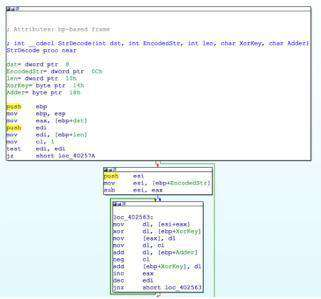An easier way to deal with this would to be implement this small algorithm as a python function and statically generate all strings from xrefs to an encoded data buffer . By using asm text kung-fu , one can extract all the parameters passed , as these functions usually do expect some constants and size parameters

``````import array

dst = array.array("B")
x = 1

for i in buf:

dst.append( (ord(i) ^ key ) & 0xff )

key = (x + adder + key) & 0xff
#print "key = %x" % key
x = x - (1 << 32) # neg in python
x = -x & 0xff
#print "x = %x" % x
#print dst.tostring()
return dst.tostring()
``````

parameter extraction can be achieved with some assembly text parsing

``````
MaxSearchStep = 5
ea  = i
while "push    " not in GetDisasm(ea):
while "push    " not in GetDisasm(ea):
#print "%x %s" % ( ea, GetDisasm(ea))
disasm = GetDisasm(ea)
while "push    " not in GetDisasm(ea):
DecStr = DecodeStr(GetManyBytes(LocByName(disasm[15:]), slen, 0), 169, 183)[0:slen].strip(" ")
print DecStr
for i in range(0, slen):
PatchByte(LocByName(disasm[15:]) + i, ord(DecStr[i])),
LocByName(disasm[15:]) + i,
MakeStr(LocByName(disasm[15:]), LocByName(disasm[15:]) + slen)
``````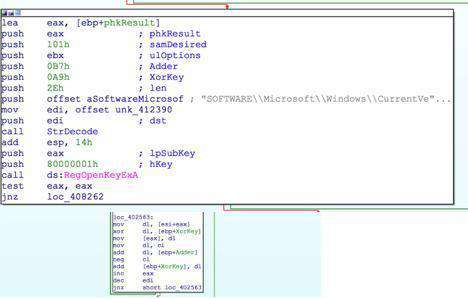This script adds a whole lot of information to the context of static analysis process

or we can leverage on the IDA APP call feature for the same result

## “Appcall is a mechanism used to call functions inside the debugged program from the debugger or your script as if it were a built-in function.” #

With APP call , we do not necessarily need to know the internal details of an underlying algorithm , but we rather call a function directly . Following example portrays a Zeus malware sample with an import table calls replaced with dynamic API retrieval calls , API call information can be retrieved using APP call on a defined function , but again by using disassembly text to extract parameters

``````
#int __fastcall get_imports(void *kernel_base, int size, int HashOfFunction, int Index);
FuncAddr = Appcall.get_imports(ECX, EDX, FirstParam, SecondParam) # API CAll
``````

Although this method works fine , but the limitations occur when parameters are not in the order we expect them to be. Due to the Turing complete nature of assembly language, parameters can be passed on in many different ways , for example

Side effects of following statements are same

``````
MOV [esp], 0xdeadbeefy (x,y two values to be added to get final value)
``````

If function has __fastcall convention then ,

``````
MOV ECX, EDX ( EDX is set earlier )
SHR ECX, 7h
PUSH 10h
POP EDX
``````

In such cases extracting parameters using text parsing would not be a viable solution .

Concolic execution in these scenarios can be of great help to retrieve such values . In Concolic execution symbols are provided with concrete values ( in our case we will provide necessary entities to reach our desired location ) , in opposite to symbolic execution which explores all paths

To help us with Concolic execution, we will make use of miasm2 library . miasm2 is a reverse engineering framework . miasm2 has its own internal IR language which is obtained from given defined assembly code . It also features an internal sandbox which can be used for run trace analysis on binaries

For illustration , we will use a sample of a Zeus variant Panda malware which does string encryption on fly using RC4 . Parameters required for a successful decrypting of string are

*1 : Four byte RC4 key
*2 : Size of string
*3 : offset to encoded data buffer
*4 : bool_convert_unicode ? ( not required)

This function happens to be __fastcall for which first two parameters are passed via registers and rest are pushed on to the stack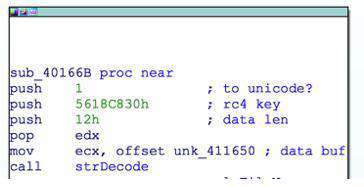Obtained parameters via xrefs and text parsing works well , but fails at a location where any parameter is retrieved indirectly . Now considering all above cases , entities can be set by many different ways .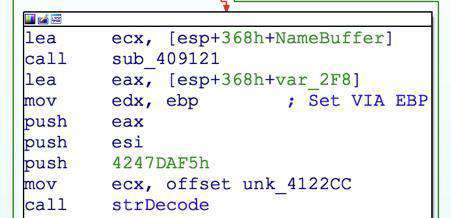In this case , EDX is retired from EBP .The value of EBP can come from different manipulations, we will be able to track via symbolic execution of this Frame

``````

from miasm2.ir.symbexec import SymbolicExecutionEngine
from miasm2.analysis.machine import Machine
from miasm2.expression.expression import ExprInt32

from miasm2.ir.symbexec import symbexec
machine = Machine('x86_32')

mdis = machine.dis_engine(bs)
start, end = 0, len(bs)
blocs = mdis.dis_multibloc(start)

ira = machine.ira()
ira.arch.regs.EAX
for bloc in blocs:
``````

Binary code of this frame till the xref of the function can be obtained by IDA API which will be processed by the library

We would also be required to provide few concrete values to the frame , as they are expected by the code to reach our desired frame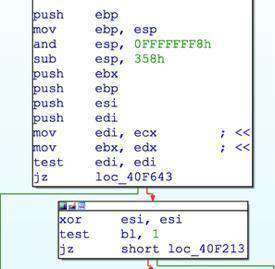Using the following code before running the symbolic engine , we will set concrete values for ECX and EDX

``````

sb.symbols[machine.mn.regs.ECX] = ExprInt32(0x0xffffffff)
sb.symbols[machine.mn.regs.EDX] = ExprInt32(0x1)
``````

And finally we let it perform symbolic execution with these concrete values

``````

sb = symbexec(ira, machine.mn.regs.regs_init)
sb.emul_ir_blocks(start)

sb.dump_id()
sb.dump_mem()
``````

This code will provide an IR dump of register and memory . It facilitates us to retrieve both the stack parameter and the register based parameters as well

``````
IRDst = 0x161
EIP = 0x161
EAX = call_func_ret(0xFFFFFF6C, call_func_stack(0xFFFF2CA3, call_func_stack(0xFFFF52AD, ((ESP_init + 0xFFFFFFFC) & 0xFFFFFFF8) + 0xFFFFFC90)) + 0xFFFFFFFC)
ECX = 0x4122CC
EDX = 0x2
EBX = 0x1
ESP = call_func_stack(0xFFFFFF6C, call_func_stack(0xFFFF2CA3, call_func_stack(0xFFFF52AD, ((ESP_init + 0xFFFFFFFC) & 0xFFFFFFF8) + 0xFFFFFC90)) + 0xFFFFFFFC)
EBP = 0x2
ESI = 0x0
EDI = 0xFFFFFFFF
zf = 0x1
nf = 0x0
pf = 0x1
of = 0x0
cf = 0x0
af = (((ESP_init + 0xFFFFFFFC) & 0xFFFFFFF8) ^ (((ESP_init + 0xFFFFFFFC) & 0xFFFFFFF8) + 0xFFFFFCA8) ^ 0x358)[4:5]
@32[call_func_stack(0xFFFF2CA3, call_func_stack(0xFFFF52AD, ((ESP_init + 0xFFFFFFFC) & 0xFFFFFFF8) + 0xFFFFFC90)) + 0xFFFFFFFC] call_func_ret(0xFFFF2CA3, call_func_stack(0xFFFF52AD, ((ESP_init + 0xFFFFFFFC) & 0xFFFFFFF8) + 0xFFFFFC90))
@32[call_func_stack(0xFFFFFF6C, call_func_stack(0xFFFF2CA3, call_func_stack(0xFFFF52AD, ((ESP_init + 0xFFFFFFFC) & 0xFFFFFFF8) + 0xFFFFFC90)) + 0xFFFFFFFC) + 0xFFFFFFFC] 0x2
@32[ESP_init + 0xFFFFFFFC] EBP_init
@32[((ESP_init + 0xFFFFFFFC) & 0xFFFFFFF8) + 0xFFFFFCA4] EBX_init
@32[((ESP_init + 0xFFFFFFFC) & 0xFFFFFFF8) + 0xFFFFFCA0] ESP_init + 0xFFFFFFFC
@32[((ESP_init + 0xFFFFFFFC) & 0xFFFFFFF8) + 0xFFFFFC9C] ESI_init
@32[((ESP_init + 0xFFFFFFFC) & 0xFFFFFFF8) + 0xFFFFFC98] EDI_init
@32[((ESP_init + 0xFFFFFFFC) & 0xFFFFFFF8) + 0xFFFFFC94] 0x0
@32[((ESP_init + 0xFFFFFFFC) & 0xFFFFFFF8) + 0xFFFFFC90] 0xD5268998
@32[((ESP_init + 0xFFFFFFFC) & 0xFFFFFFF8) + 0xFFFFFC8C] 0x7
``````

From this output , we can retrieve our required parameters which happen to be   EDX = 0x2 : Length of string
ECX = 0x4122CC : Address of encoded data buffer

@32[((ESP_init + 0xFFFFFFFC) & 0xFFFFFFF8) + 0xFFFFFC94] 0x0 *: bool_to_unicode ?
*@32[((ESP_init + 0xFFFFFFFC) & 0xFFFFFFF8) + 0xFFFFFC90] 0xD5268998
: RC4Key

Backtracking reveals the location where EBP was set to 2, which was traced and recorded by symbolic engine till the end of frame